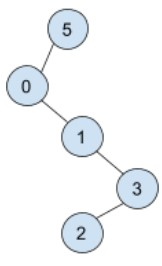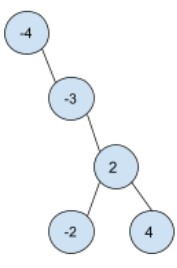New update is available. Click here to update.
Topics

# Two Sum IV - Input is a BST

Moderate0/80
0 upvote## Problem statement

You are given a binary search tree consisting of ‘N’ nodes. Each node has a weight associated with it. Ninja recently learned about tree algorithms and the teacher wants him to know whether a pair of nodes exists whose sum of values is exactly equal to ‘K’.

Output true if such a pair exists.

Detailed explanation ( Input/output format, Notes, Images )
Constraints :
``````1 <= T <= 10
1 <= N <= 10^4
-10^4 <= value of node[i] <= 10^4
Value of node[i] is never equal to -1
It is guaranteed that the given input is a binary tree.

Time Limit: 1 sec
``````
Sample Input 1 :
``````2
5 3
5 0 -1 -1 1 -1 3 2 -1 -1 -1
5 -6
-4 -1 -3 -1 2 -2 4 -1 -1 -1 -1
``````
Sample Output 1 :
``````true
true
``````
Explanation Of Sample Input 1 :
``````For test case 1 we have,

The input tree:
````````````The pair of values {0, 3} have sum 3.

So, we output 1(true).

For test case 2 we have,

The input tree :
````````````The pair of values {-4, -2} have sum -6.

So, we output 1(true).
``````
Sample Input 2 :
``````2
4 8
4 -2 -1 -3 3 -1 -1 -1 -1
3 4
5 0 -1 -4 -1 -1 -1
``````
Sample Output 2 :
``````true
false
``````Console